## What is the difference between 5/6 and 1/5

Question

What is the difference between 5/6 and 1/5

in progress 0
7 months 2021-08-01T17:04:37+00:00 1 Answers 5 views 0

1. Hey there!

The answer is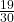To solve this we use subtraction, because “the difference” represents subtraction, so: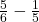Now, we must find a common denominator, and we can see that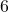and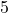both go into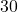. To get to, we multiplied the first fraction by 5 and the second by 6, so we now have: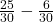This is equal toHope it helps and have a great day!